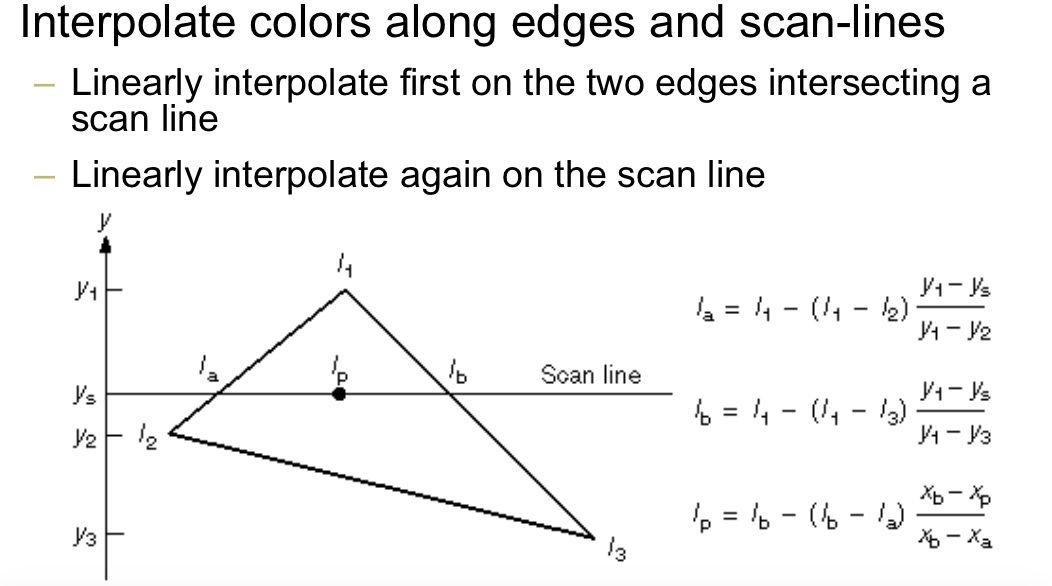Shading refers to depicting depth perception in 3D models or illustrations by varying levels of darkness. In computer graphics, shading refers to the process of altering the color of an object in 3D scene, based on its angle to lights and its distance from lights to create a photorealistic effect.

• compute illumination once per polygon and apply it to whole polygon or take color of first vertex
• compute illumination at boundary and interpolate over the interior
• compute illumination at every point of the polygon
• Intensity discontinuity across edge
• If there are very large number of very small polygons, the faceting effects is not obvious
• Interpolative
• Given colors of the polygn vertices, interior points are colored through bilinear interpolation
• Vertex normals vs. Face Normals
• Use the actual surface’s normal at each vertex, when the surface is not analytically defined, take the average normal of all neighboring faces• “Color computation only at vertices”
• illumination mode evaluation at vertex shader
• Simple interpolation at fragment shader
• Fast and easy
• “Color computation only at vertices”
• Does not simulate the